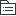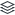# 挖机装配

7
|
2

## 相关模板推荐

•## 挖掘机 —— 作品大纲

•上车组装
• 底板装配
• 底板分装
• 脚踏板阀安装
• 各胶管管路安装
• 行走操纵装置的安装
• 液压启动操纵装置
• 座椅导轨
• 空调面板支架总成
• 左右操纵箱的安装
• 左右液压先导阀总成
• 空调蒸发其部件的安装
• 法兰及保护罩的安装
• 布线布管
• 回转平台清丝
• 电器管路安装
• 空调管路安装
• 柴油管路安装
• 回转马达安装
• 驾驶室装配
• 座椅安装
• 空调罩总成
• 驾驶室安装
• 驾驶室分装
• 卸载驾驶室内饰件
• 压线 加插头
• 安装驾驶室顶灯
• 喷淋水壶组装
• 主阀总成装配
• 主阀分装
• 安装阀芯
• 安装胶管
• 安装钢管
• 安装左右支架
• 液压件装配
• 回转马达管接头安装
• 回转马达先导管和回油管安装
• 回油阀部装
• 回油阀安装
• 连接先导管
• 防水板安装
• 动臂油缸主管路安装
• 回转马达回油管安装
• 发动机总装
• 减震系统的安装
• 发动机总成安装
• 发动机分装
• 主泵安装
• 联轴器安装
• 安装接头连接胶管
• 分装空调压缩机
• 安装排气管
• 安装排水管
• 安装机油接口安装机油管
• 散热器总成的安装
• 冷凝器的安装
• 空调管路的安装
• 进排气管的安装
• 固定机油管
• 熄火装置的安装
• 油门机构的安装
• 进出水管的安装
• 油箱装配
• 油箱附件的安装
• 燃油箱液压油箱的安装
• 液压系统吸油管的安装
• 回油系统装配
• 软性接头的安装
• 散热回油钢管的安装
• 管接头的安装
• 隔板装配
• 支撑横梁安装
• 左右门安装
• 隔板安装
• 系统件安装
• 覆盖件总装
• 盖板安装
• 空滤器管道安装
• 间隙检查
• 燃油系统装配
• 过滤器的安装
• 储液器的安装
• 滤油器的安装
• 管路的安装
• 工具箱机顶罩装配
• 机顶罩的安装
• 工具箱的安装
• 蓄电池的安装
• 工作灯的安装
• 加油加水
• 柴油、液压油、防冻液的加注
•下车组装
• 支重轮装配
• 装配前检查
• 安装支撑轮
• 安装护板
• 翻转
• 托链轮装配
• 装配前检查
• 托链轮装配
• 回转接头导向装配
• 螺纹口清丝
• 安装导向装置总成
• 吊装回转接头和安装过度板
• 固定回转接头
• 行走马达装配
• 螺纹孔全部清丝
• 行走马达安装面上的防锈剂清理
• 安装减速机
• 安装驱动轮
• 安装马达
• 行走管路连接
• 安装护套
• 安装管接头 打力矩
• 安装法兰片，螺钉，打力矩
• 固定胶管
• 回转支撑装配
• 安装面清洗，涂胶
• 安装密封组件
• 安装回转支承
• 安装密封垫，方接头
• 安装硬管
• 安装油杯
• 转下道工序
• 履带装配
• 打卡履带
• 放履带架
• 起吊履带一头
• 吊压销工装，压销
• 加润滑脂
• 判定间隙
•合车
• 合车
• 合车装配
• 回转接头先导管
• 回油管安装
• 动臂油缸的安装
• 动臂总装
• 动臂分装
• 动臂管路装配
• 斗杆总装
• 斗杆分装
• 清洁各部件
• 轴套的压装
• 斗杆油缸安装
• 管路安装
• 斗杆管路装配
• 斗杆部装总成吊装
• 斗杆根部销轴安装
• 斗杆油缸连接
• 铲斗总装
• 铲斗总成吊装
• 销轴安装
• 铲斗 连杆对接
• 铲斗分装
• 清洁各部件
• 轴套的压装
• 铲斗油缸安装
• 连杆和摇杆的安装
• 管路的安装
• 配重装配
• 降噪海绵安装
• 配重装配
• 间隙调整
• 下线调试
•油缸组装
• 动臂油缸分装
• 安装动臂油缸管卡组件（左右）
• 安装动臂油缸钢管
• 斗杆油缸分装
• 安装铲斗油缸钢管
• 安装铲斗油缸钢管管卡组件
• 安装铲斗油缸
• 安装铲斗油缸钢管
• 安装铲斗油缸管卡组件